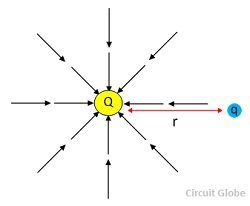# Electric Field

Definition: The region around the electric charge in which the stress or electric force act is called an electric field or electrostatic field. If the magnitude of charge is large, then it may create a huge stress around the region. The electric field is represented by the symbol E. The SI unit of the electric field is newton per coulomb which is equal to volts per meter.The electric field is represented by the imaginary lines of force. For the positive charge, the line of force come out of the charge and for negative charge the line of force will move towards the charge. The electric field for positive and negative charges are shown belowConsider a unit charge Q placed in a vacuum. If another charge q is placed near the Q then according to Coulomb law, the charge Q apply a force on it. The charge Q produce an electric field around it, and when any other charge is placed near it, then the electric field of Q apply force on it.The electric field produced  by the charge Q at a point r is given bywhere Q – unit charge
r – distance between the charges

A charge Q applies the force on a charge q is expressed byThe charge q also apply an equal and opposite force on the charge Q.

## Types of an Electric Field

The electric field is mainly classified into two types. They are the uniform electric field and the nonuniform electric field.

## 1. Uniform Electric Field

When the electric field is constant at every point, then the field is called the uniform electric field. The constant field is obtained by placing the two conductor parallel to each other, and the potential difference between them remains same at every point.

##2. Non-Uniform Electric Field

The field which is irregular at every point is called the non-uniform electric field. The non-uniform field has a different magnitude and directions.

###Properties of an Electric Field

The following are the properties of an electric field.

1. Field lines never intersect each other.
2. They are perpendicular to the surface charge.
3. The field is strong when the lines are close together, and it is weak when the field lines move apart from each other.
4. The number of field lines is directly proportional to the magnitude of the charge.
5. The electric field line starts from the positive charge and ends from negative charge.
6. If the charge is single, then they start or end at infinity.
7. The line curves are continuous in a charge-free region.

When the electric and magnetic field combines, they form the electromagnetic field.

### 11 thoughts on “Electric Field”

1. abhijeet jha jr

field lines are imaginary so there is no question of attraction between field lines.